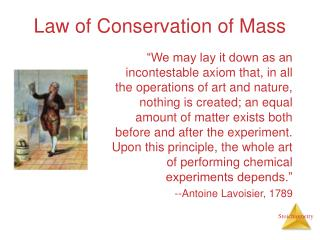# Law of Conservation of Mass - PowerPoint PPT PresentationDownload PresentationLaw of Conservation of Mass

Law of Conservation of Mass
Download Presentation## Law of Conservation of Mass

- - - - - - - - - - - - - - - - - - - - - - - - - - - E N D - - - - - - - - - - - - - - - - - - - - - - - - - - -
##### Presentation Transcript

1. Law of Conservation of Mass “We may lay it down as an incontestable axiom that, in all the operations of art and nature, nothing is created; an equal amount of matter exists both before and after the experiment. Upon this principle, the whole art of performing chemical experiments depends.” --Antoine Lavoisier, 1789

2. Reaction Types

3. Examples: N2 (g) + 3 H2 (g) 2 NH3 (g) C3H6 (g) + Br2 (l)  C3H6Br2 (l) 2 Mg (s) + O2 (g)  2 MgO (s) Two or more substances react to form one product Combination Reactions

4. 2 Mg (s) + O2 (g)  2 MgO (s)

5. Examples: CaCO3 (s) CaO (s) + CO2 (g) 2 KClO3 (s)  2 KCl (s) + O2 (g) 2 NaN3 (s) 2 Na (s) + 3 N2 (g) One substance breaks down into two or more substances Decomposition Reactions

6. Examples: CH4 (g) + 2 O2 (g) CO2 (g) + 2 H2O (g) C3H8 (g) + 5 O2 (g)  3 CO2 (g) + 4 H2O (g) Rapid reactions that produce a flame Most often involve hydrocarbons reacting with oxygen in the air Combustion Reactions

7. Mole Relationships • One mole of atoms, ions, or molecules contains Avogadro’s number of those particles • One mole of molecules or formula units contains Avogadro’s number times the number of atoms or ions of each element in the compound

8. Finding Empirical Formulas

9. Calculating Empirical Formulas One can calculate the empirical formula from the percent composition

10. Calculating Empirical Formulas The compound para-aminobenzoic acid (you may have seen it listed as PABA on your bottle of sunscreen) is composed of carbon (61.31%), hydrogen (5.14%), nitrogen (10.21%), and oxygen (23.33%). Find the empirical formula of PABA.

11. Assuming 100.00 g of para-aminobenzoic acid, C: 61.31 g x = 5.105 mol C H: 5.14 g x = 5.09 mol H N: 10.21 g x = 0.7288 mol N O: 23.33 g x = 1.456 mol O 1 mol 12.01 g 1 mol 14.01 g 1 mol 16.00 g 1 mol 1.01 g Calculating Empirical Formulas

12. Calculate the mole ratio by dividing by the smallest number of moles: C: = 7.005  7 H: = 6.984  7 N: = 1.000 O: = 2.001  2 5.09 mol 0.7288 mol 0.7288 mol 0.7288 mol 5.105 mol 0.7288 mol 1.458 mol 0.7288 mol Calculating Empirical Formulas

13. Calculating Empirical Formulas These are the subscripts for the empirical formula: C7H7NO2

14. Combustion Analysis • Compounds containing C, H and O are routinely analyzed through combustion in a chamber like this • C is determined from the mass of CO2 produced • H is determined from the mass of H2O produced • O is determined by difference after the C and H have been determined

15. Stoichiometric Calculations The coefficients in the balanced equation give the ratio of moles of reactants and products

16. Stoichiometric Calculations From the mass of Substance A you can use the ratio of the coefficients of A and B to calculate the mass of Substance B formed (if it’s a product) or used (if it’s a reactant)

17. Stoichiometric Calculations C6H12O6 + 6 O2 6 CO2 + 6 H2O Starting with 1.00 g of C6H12O6… we calculate the moles of C6H12O6… use the coefficients to find the moles of H2O… and then turn the moles of water to grams

18. Limiting Reactants

19. How Many Cookies Can I Make? • You can make cookies until you run out of one of the ingredients • Once this family runs out of sugar, they will stop making cookies (at least any cookies you would want to eat)

20. How Many Cookies Can I Make? • In this example the sugar would be the limiting reactant, because it will limit the amount of cookies you can make

21. Limiting Reactants • The limiting reactant is the reactant present in the smallest stoichiometric amount • In other words, it’s the reactant you’ll run out of first (in this case, the H2)

22. Limiting Reactants In the example below, the O2 would be the excess reagent